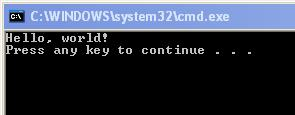## Monday, January 6, 2014

### For loops and while loops in c#

The loops are used for repeating a statement or group of statement.

For Loop:
We need the following,to use this loop.
1.A starting value to initialize the loop.
2.A condition for running the loop.
3.An operation to update the loop.

Example:

static void Main(string[]args)
{
int i, num,sum;
sum=0;
for(i = 0; i < 5; i ++)
{
Console.WriteLine("Enter a number");
sum = sum + num;
}
Console.WriteLine("The sum of all the numbers is {0}", sum);
}

While Loop
The while loop is almost similar to the 'for loop' with some difference in the syntax.

Example:

static void Main(string[]args)
{
int i, num, sum;
sum = 0;
i =0;
while(i < 5)
{
Console.WriteLine("Enter a number");
sum = sum + num; i ++;
}
Console.WriteLine("The sum of all the numbers is {0}", sum);
}

### C# switch-case statement

The switch-case statement is also use for making decision. However, unlike the if-else statement,we can't test conditions like "greater than" or "less than". In a switch-case, we can test the value of a variable and decide what to do if a particular value is stored in the variable.
Example:

switch(variable to test)
{
case value1:
statements if value1 stored in the variable;
break;

case value2:
statements if value12 stored in the varible;
break;

default:
statements if none of the values match:
break;
}

Example:
The use will enter a weekday number (1 to 7) and the program will display the name of the corresponding day:

static void Main(string[]args)
{
int day:
Console.WriteLine("Enter a weekday number (1-7)");
{
case1:Console.WriteLine("Sunday");
break;
case2:Console.WriteLine("Monday");
break;
case3:Console.WriteLine("Tuesday");
break;
case4:Console.WriteLine("Wednesday");
break;
case5:Console.WriteLine("Thursday");
break;
case6:Console.WriteLine("Friday");
break;
case7:Console.WriteLine("Saturday");
break;
}
}

## Saturday, January 4, 2014

### C# if - else statement

// Try this code

int num;
Console.WriteLine("Enter a number");
if(num % 2 == 0)
{
Console.WriteLine("The number is even");
}
else
{
Console.WriteLine("The number is odd");
}

// Test multiple conditions using the if-else statement

if(condition!)
{
statements if the condition 1 is true;
}
else if (condition 2)
{
statements if the condition 2 is true;
}
else
{
statement if none of the condition are true;
}

### Increment and Decrement in C#

There are two operators use for increasing or decreasing the values of a variable by 1.
The increment operator is denote by "++" and the decrement operator is denoted by "--".

Example

int num;
num =10;
num++;

After the last statement, the value of 'num' is 11.Similarly,

int num;
num=10;
num--;

The last statement will decrease the value of  'num' by 1 and make it 9.

### C# symbol operator

Operator             Use

-                         Substration
*                        Multiplication
/                         Division
%                       Reminder of division

Logical operators.They compare two values and give result in 'true' or 'false'.
They are mostly used with the decision making statements.

Operator              Use

= =                       Equal
! =                        Not equal
>                          Greater than
<                          Less than
>=                        Greater than or not equal to
<=                        Less than or not equal to

&&                      Check multiple conditions. If all the conditions are true, the result is 'true'. If any one
condition is false, the result is 'false'.

||                           If any on of the conditions is true, the result is 'true'. If all the conditions are false, the
result is 'false'.

### Data types and variables in c#

Data Type: A data type is specifies whether a variable can store a number, a single character or text.

Variables are names given to the memory location for string data. Data Types are used to some commonly use data types in C# are:

Data Type          Use

int                      For storing integer values
long                   Storing large integer values
float                   storing floating point values
double               storing large floating point values
char                   storing a single character
string                 storing a string
bool                  storing the values 'true' or 'false'

Examples:
int salary;
int number1,number2;
string name;
int age;

C-Sharp tutorials for beginners. It is possible to make a project with this article.

//Open the Visual C#
//click the new project
//console application
//type the name of project

```using System;
using System.Collections.Generic;
using System.Text;

namespace MyFirstApplication
{
class Program
{
static void Main(string[] args)
{
Console.WriteLine("Hello, world!");
}
}```

### Running the Program

On the Debug menu, click Start Without Debugging (or just press Ctrl-F5). A command prompt window appears that looks like this:
` `
``
` `